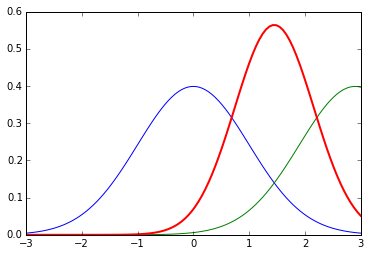# Interactive: Cue Combination¶

This demo shows how cues combine weighted by their uncertainty as their variances change.

In :
%matplotlib inline
import matplotlib.pyplot as plt
import numpy as np
import matplotlib.mlab as mlab
import math

def plotGaussian(mean, std, color, width):
x = np.linspace(-3,3,100)
plt.plot(x,mlab.normpdf(x,mean,std), color, linewidth=width)

def CueIntegration(mean1=0, mean2=1, std1=2, std2=1):
plotGaussian(mean1, std1, 'b', 1)
plotGaussian(mean2, std2, 'g', 1)
mean = mean2 * (std1**2 / (std1**2+std2**2)) + mean1 * (std2**2 / (std1**2+std2**2))
std = (std1 * std2) / np.sqrt(std1 ** 2 + std2 ** 2)
plotGaussian(mean, std, 'r', 2)

from IPython.html.widgets import interact
interact(CueIntegration, mean1=(0,4,0.1), mean2=(0,4,0.1), std1=(0.1,4,0.1), std2=(0.1,4,0.1))